Shipra Saxena — March 16, 2021

## Objective

• LSTM is a special kind of recurrent neural network capable of handling long-term dependencies.
• Understand the architecture and working of an LSTM network

## Introduction

Long Short Term Memory Network is an advanced RNN, a sequential network, that allows information to persist. It is capable of handling the vanishing gradient problem faced by RNN. A recurrent neural network is also known as RNN is used for persistent memory.

Let’s say while watching a video you remember the previous scene or while reading a book you know what happened in the earlier chapter. Similarly RNNs work, they remember the previous information and use it for processing the current input. The shortcoming of RNN is, they can not remember Long term dependencies due to vanishing gradient. LSTMs are explicitly designed to avoid long-term dependency problems.

Note: If you are more interested in learning concepts in an Audio-Visual format, We have this entire article explained in the video below. If not, you may continue reading.

## LSTM Architecture

At a high-level LSTM works very much like an RNN cell. Here is the internal functioning of the LSTM network. The LSTM  consists of three parts, as shown in the image below and each part performs an individual function.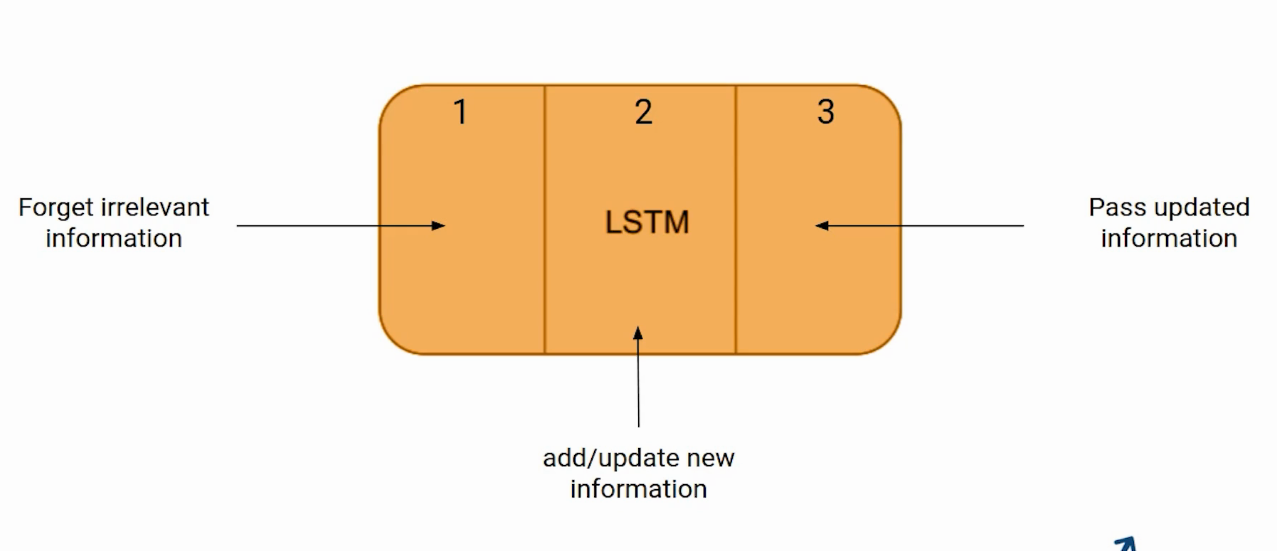The first part chooses whether the information coming from the previous timestamp is to be remembered or is irrelevant and can be forgotten. In the second part, the cell tries to learn new information from the input to this cell. At last, in the third part, the cell passes the updated information from the current timestamp to the next timestamp.

These three parts of an LSTM cell are known as gates. The first part is called Forget gate, the second part is known as the Input gate and the last one is the Output gate.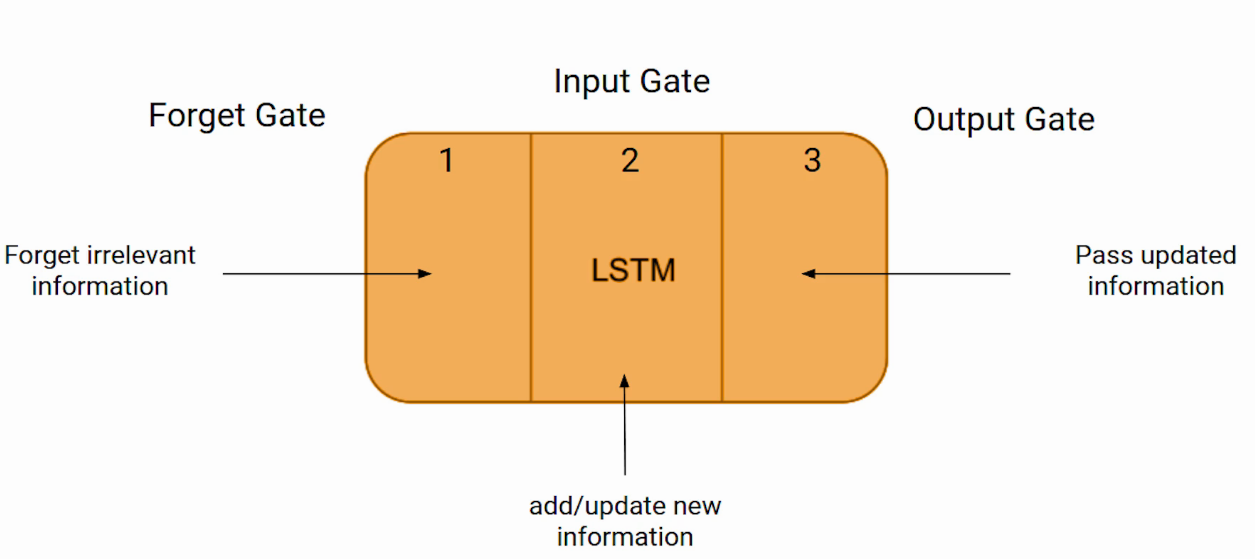Just like a simple RNN, an LSTM also has a hidden state where H(t-1) represents the hidden state of the previous timestamp and Ht is the hidden state of the current timestamp. In addition to that LSTM also have a cell state represented by C(t-1) and C(t) for previous and current timestamp respectively.

Here the hidden state is known as Short term memory and the cell state is known as Long term memory. Refer to the following image.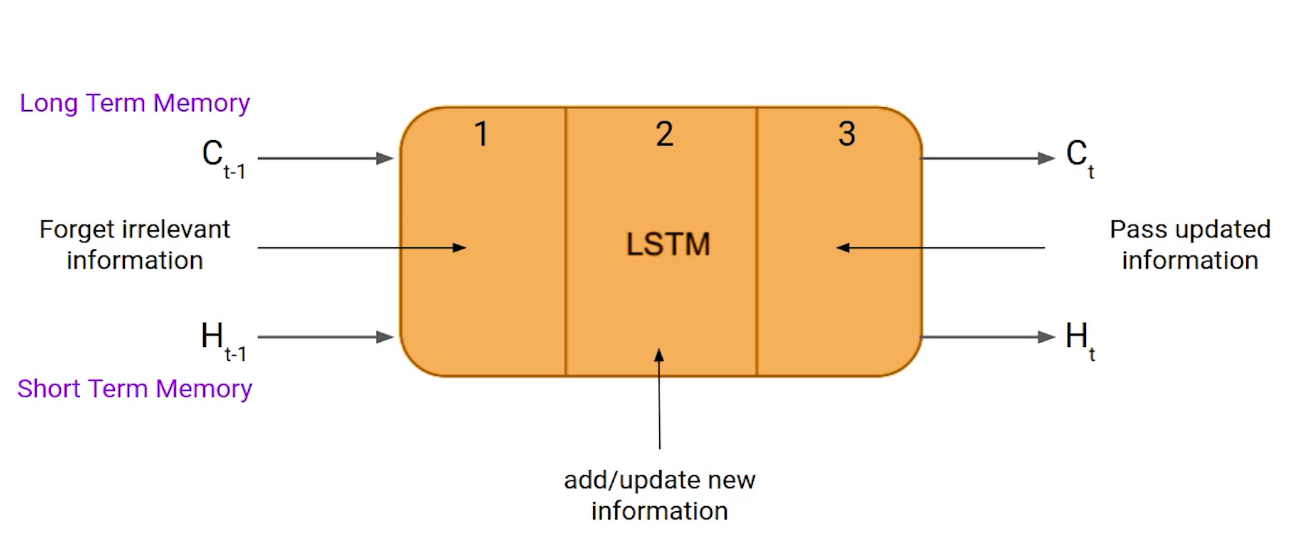It is interesting to note that the cell state carries the information along with all the timestamps.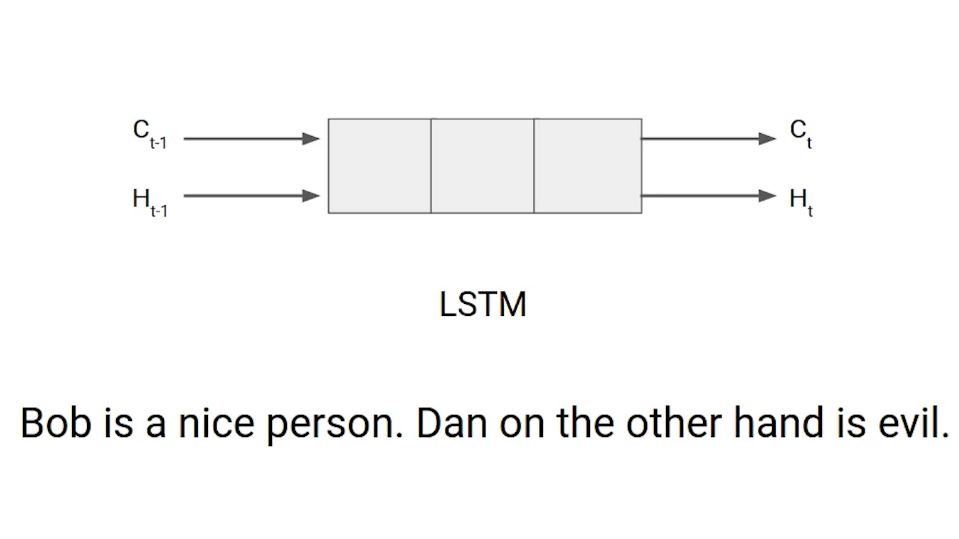Let’s take an example to understand how LSTM works. Here we have two sentences separated by a full stop. The first sentence is “Bob is a nice person” and the second sentence is “Dan, on the Other hand, is evil”. It is very clear, in the first sentence we are talking about Bob and as soon as we encounter the full stop(.) we started talking about Dan.

As we move from the first sentence to the second sentence, our network should realize that we are no more talking about Bob. Now our subject is Dan. Here, the Forget gate of the network allows it to forget about it. Let’s understand the roles played by these gates in LSTM architecture.

### Forget Gate

In a cell of the LSTM network, the first step is to decide whether we should keep the information from the previous timestamp or forget it. Here is the equation for forget gate.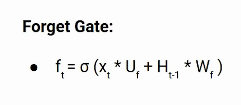Let’s try to understand the equation, here

• Xt: input to the current timestamp.
• Uf: weight associated with the input
• Ht-1: The hidden state of the previous timestamp
• Wf: It is the weight matrix associated with hidden state

Later, a sigmoid function is applied over it. That will make ft a number between 0 and 1. This ft is later multiplied with the cell state of the previous timestamp as shown below.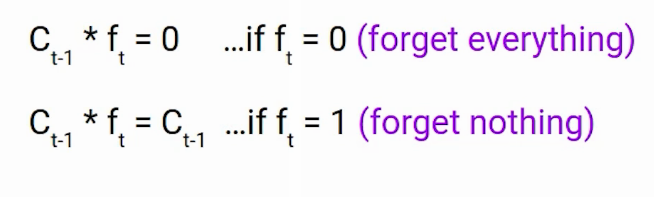If ft is 0 then the network will forget everything and if the value of ft is 1 it will forget nothing. Let’s get back to our example, The first sentence was talking about Bob and after a full stop, the network will encounter Dan, in an ideal case the network should forget about Bob.

### Input Gate

Let’s take another example

“Bob knows swimming. He told me over the phone that he had served the navy for four long years.”

So, in both these sentences, we are talking about Bob. However, both give different kinds of information about Bob. In the first sentence, we get the information that he knows swimming. Whereas the second sentence tells he uses the phone and served in the navy for four years.

Now just think about it, based on the context given in the first sentence, which information of the second sentence is critical. First, he used the phone to tell or he served in the navy. In this context, it doesn’t matter whether he used the phone or any other medium of communication to pass on the information. The fact that he was in the navy is important information and this is something we want our model to remember. This is the task of the Input gate.

Input gate is used to quantify the importance of the new information carried by the input. Here is the equation of the input gate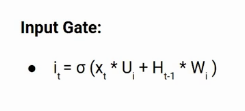Here,

• Xt: Input at the current timestamp t
• Ui: weight matrix of input
• Ht-1: A hidden state at the previous timestamp
• Wi: Weight matrix of input associated with hidden state

Again we have applied sigmoid function over it. As a result, the value of I at timestamp t will be between 0 and 1.

### New information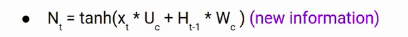Now the new information that needed to be passed to the cell state is a function of a hidden state at the previous timestamp t-1 and input x at timestamp t. The activation function here is tanh. Due to the tanh function, the value of new information will between -1 and 1. If the value is of Nt is negative the information is subtracted from the cell state and if the value is positive the information is added to the cell state at the current timestamp.

However, the Nt won’t be added directly to the cell state. Here comes the updated equation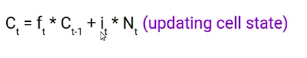Here, Ct-1 is the cell state at the current timestamp and others are the values we have calculated previously.

### Output Gate

Now consider this sentence

“Bob single-handedly fought the enemy and died for his country. For his contributions, brave________ .”

During this task, we have to complete the second sentence. Now, the minute we see the word brave, we know that we are talking about a person. In the sentence only Bob is brave, we can not say the enemy is brave or the country is brave. So based on the current expectation we have to give a  relevant word to fill in the blank. That word is our output and this is the function of our Output gate.

Here is the equation of the Output gate, which is pretty similar to the two previous gates.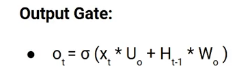Its value will also lie between 0 and 1 because of this sigmoid function. Now to calculate the current hidden state we will use Ot and tanh of the updated cell state. As shown below.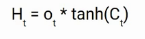It turns out that the hidden state is a function of Long term memory (Ct) and the current output.  If you need to take the output of the current timestamp just apply the SoftMax activation on hidden state Ht.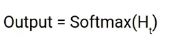Here the token with the maximum score in the output is the prediction.

This is the More intuitive diagram of the LSTM network.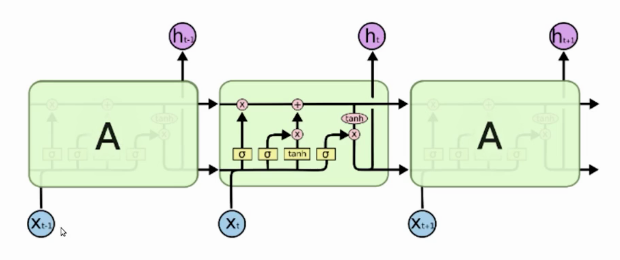This diagram is taken from an interesting blog. I urge you all to go through it. Here is the link-

## End Notes

To summarize, in this article we saw the architecture of a sequential model LSTM and how it works in detail.

If you are looking to kick start your Data Science Journey and want every topic under one roof, your search stops here. Check out Analytics Vidhya’s Certified AI & ML BlackBelt Plus Program

If you have any questions, let me know in the comments section!